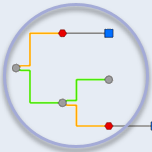Network Diagrams Team

The Network Diagrams Team blogs about network diagram topics. #UtilityNetwork #TraceNetwork #EsriElectric #EsriGas

Posts by this author
Porting ArcGIS Desktop Schematic Diagrams to ArcGIS Pro Network Diagrams

Learn how to port schematic diagrams created with ArcGIS Schematics to network diagrams from utility or trace networks using ArcGIS Pro

How network diagram consistency states switch during a workflow

Learn about network diagram consistency states and how they switch for standard diagrams and subnetwork system diagrams during your workflow.

A tale of two network diagrams: Subnetwork system diagrams and standard diagrams

Learn about differences between subnetwork system diagrams and standard diagrams to decide whether system diagrams are relevant for you

How do utility or trace network changes impact network diagrams?

Learn about how changes on utility network or trace network features impact network diagrams and what can be done next

Editing in network diagrams

Learn about diagram feature geometries and how you can edit your network diagram contents